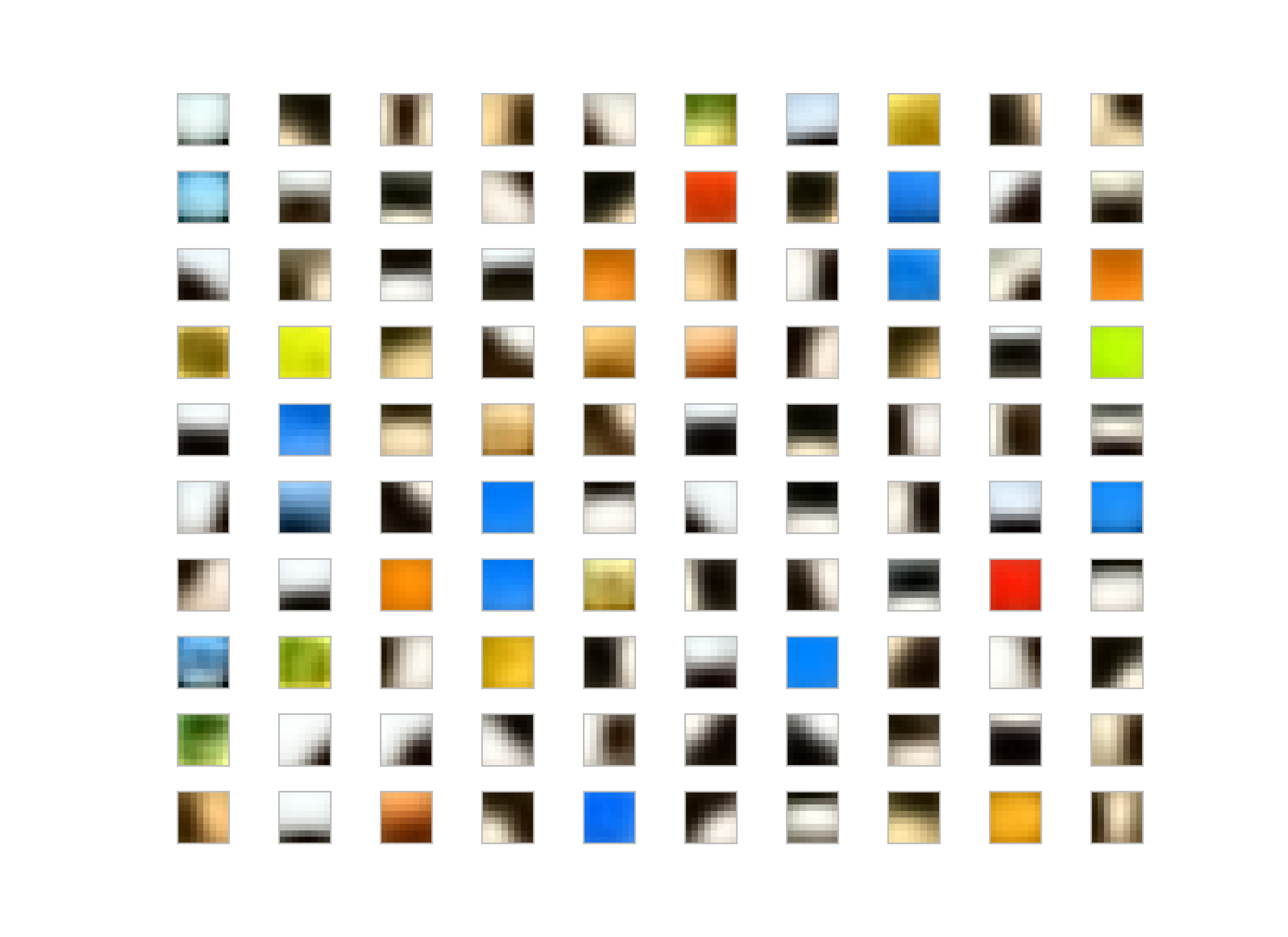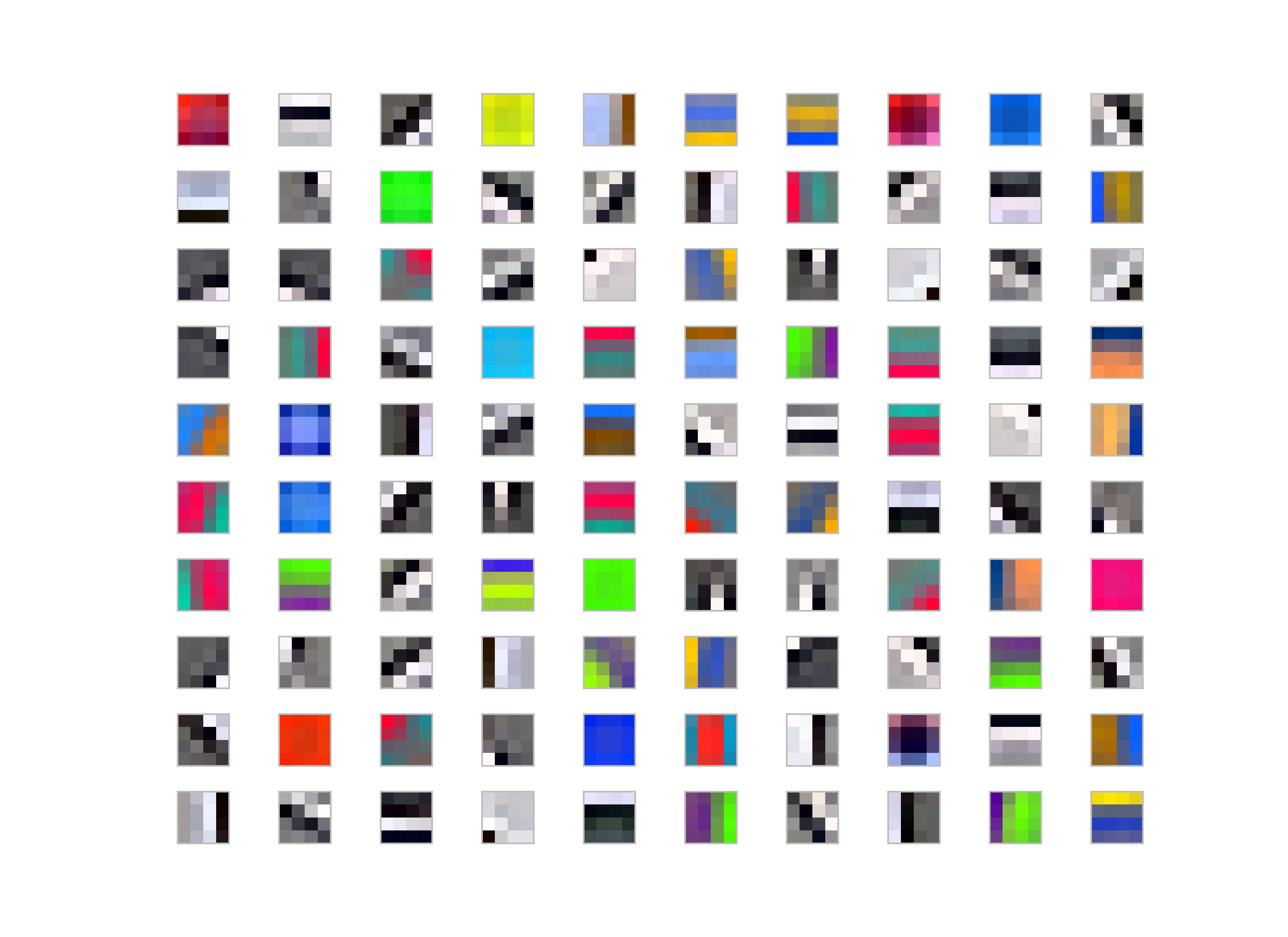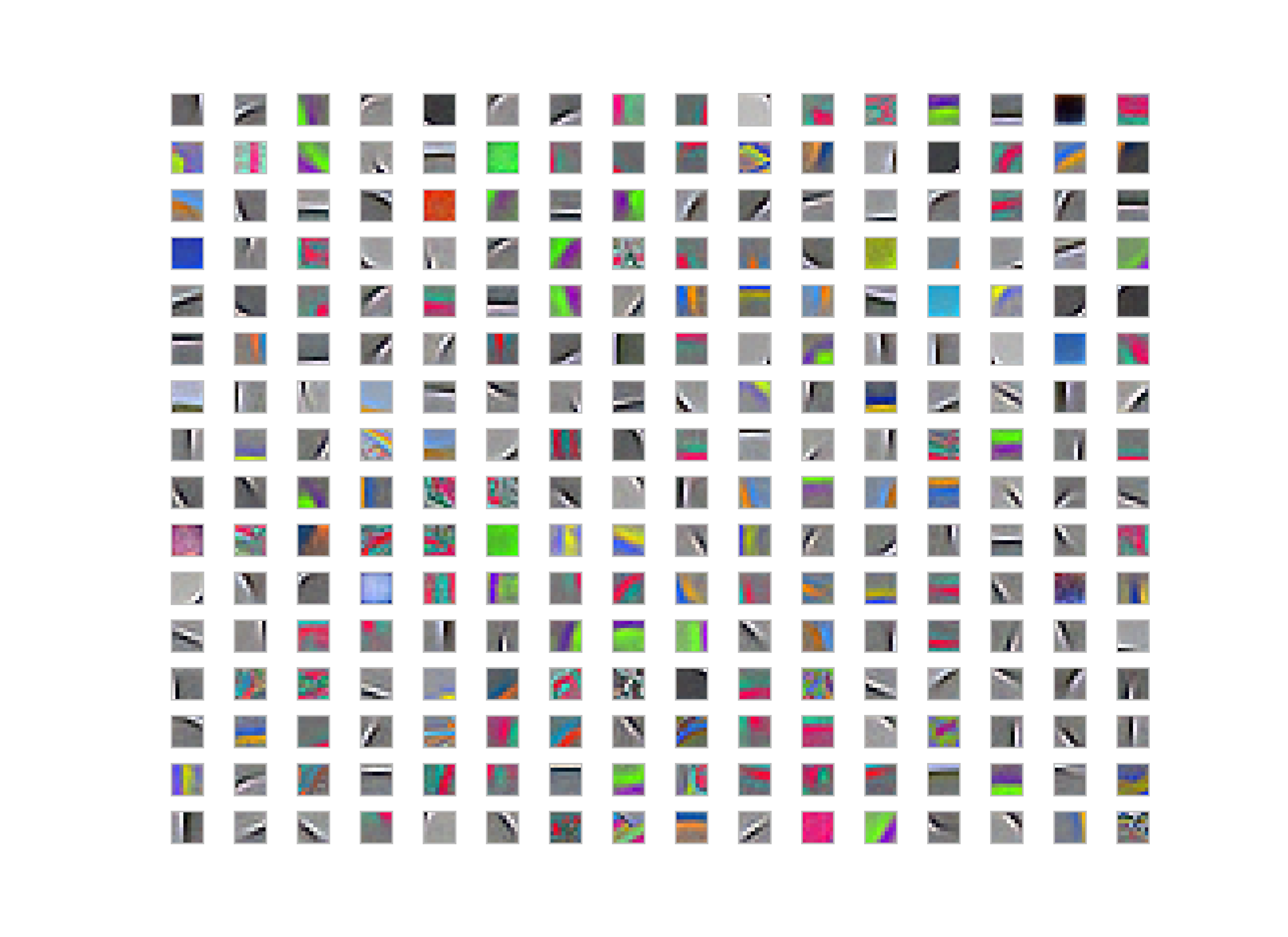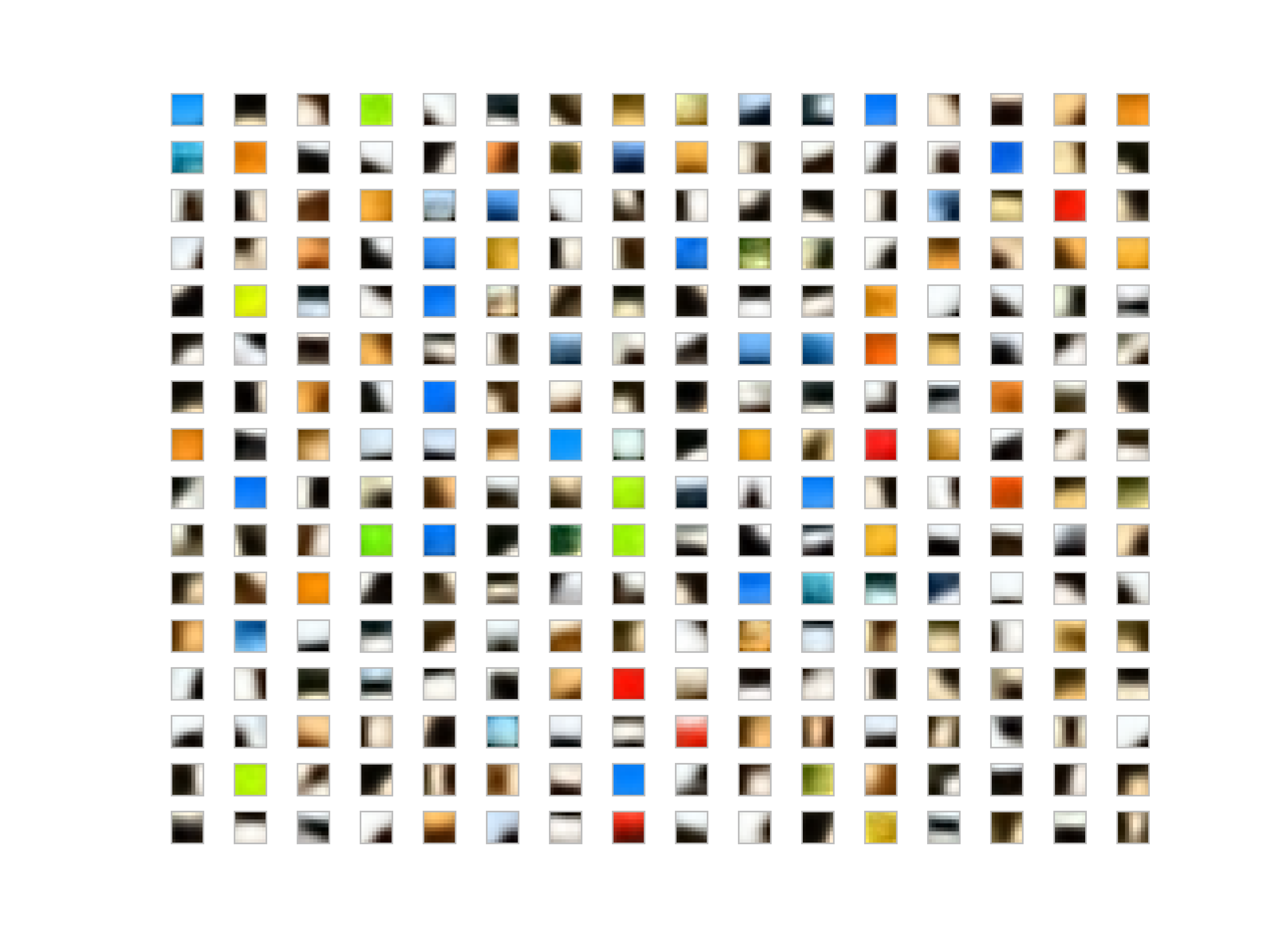# K-Means, Sparse Coding, Dictionary Learning and All That

February 10, 2015 · -

### Feature Learning

Feature learning especially from images is a fundamental step in a classification pipeline as the raw pixels create both huge feature vectors per image and are not necessarily good to represent the image in an efficient way for image classification problems.

### Unsupervised Feature Learning

Computer vision community came up with engineered feature detectors first (SIFT, HoG and so on), then try to use unsupervised learning algorithm to find useful visual “word”s in the image so that they could be used for the classification. Nowadays, deep learning is used for feature extraction and also classification at some extent. However, one thing did not change; to be able to learn important and relevant features from the images in an automated way.

Adam Coates in Andrew Ng’s group put a lot of work in this area. The premise is quite exciting, to be able to learn features similar to sparse coding or dictionary learning using one of the simplest and efficient algorithm(k-means, surprise!) which is easily scalable and parallelizable(a variant of k-means albeit).

### Experiments

I used CIFAR 100 datasets to learn and display the filters. Sadly, I could not get better than what papers reported so I’ll skip on reporting the classification accuracies. Every section will have the filters that are learned in the CIFAR dataset so that you could also have chance to observe similarities and differences.

### K-Means

K-Means could be one of the most commonly used unsupervised learning algorithm both it is easy to implement, efficient and also it is easy to reason about. Basic premise of the K-Means boils down to represent the observations in a much efficient representation where some observations are mapped to a cluster points.

### K-Means Problem Formulation

There are $n$ observations given a dataset $P$ where we represent the samples as follows:

$P = {x^{(i)}}_{i=1}^n \subseteq R^d$ where we want to represent the samples in a number of clusters $k \in N$.(so the k-means name)

### K-Means Objective Function

We want to find $k$ number of centroids ${c^{1}, , c^{(k)}} R^d$, which are the local(global minimum is intractable) minimum of the following objective function: $B_P(c) = \frac{1}{n}\displaystyle\sum_{i=1}^n \min_{j \in k} \lVert x^{(i)} - c^{(j)} \rVert_2^2$

Most common algorithm is to use an EM(Expectation Maximization Algorithm); first change the centroids position(or randomly initialize) and then compute the distance of the observations to the centroids and then reassign the observations to the closest centroids. After a number of iterations, either centroids would not move at all, or their distance change would be minimal, so the convergence.

### K-Means Triangle

There is a modified version of K-Means which is called K-Means Tri where every observation does not get the hard cluster assignments(1 or 0) but rather a score within each cluster. This creates a competition in the features inside of the cluster. This allows each feature to “compete” to get the highest score. This could be much more accurate for the clusters that are quite large where the all of the observations get same score. Empirically, it also produces sparse outputs as well.

### K-Means Tri Objective Function

Objective function does not change but the scores of each observation changes. $f_k(x) = \max(0, \mu(z) - z_k)$ where $z_k=x - c^{k} _2$ and $\mu(z)$ is the mean of observations in $z$.

### K-Means Results

I run the K-Means Tri with whitening and without whitening on the dataset. My observation, for the K-Means without whitening is the patches end up low-frequency and color dominant whereas the whitened patches are more like edge-like filters.

### Patch Length = 8

Whitened filters:K-Means Filters - Unnormalized with Stride=8

Not-whitened filters:K-Means Filters - Unnormalized with Stride=8

### Patch Length = 4

Whitened filters:K-Means Filters with Stride=4

Not-whitened filters:K-Means Filters with Stride=4

Generally people use some whitening for various reasons; for K-Means, it makes easier to converge also filters-learned are more edge-like rather than pure colors or low-frequency parts in the image as you could see the result of the images given above.

### Sparse Coding

Sparse Coding like most of the decomposition/factorization methods try to represent a signal with different atoms, in the case of sparse coding, these atoms are sparse and the matrix that yields this atom is overcomplete. This sparsity and overcompleteness nature of the matrix enables a robust reconstruction of the signal from very few atoms as long as some conditions are met.

Representation of image could be done by linear transform in the following way:

$x = \displaystyle\sum_{i=1}^m a_i \phi_i$ where $m n$ so that we have an overcomplete representation of the original observation matrix.

Sparse coding and most of the atom based methods try to get a variety of atoms either it is a precomputed matrix(FFT, PCA, etc) or a “dictionary” as in the dictionary learning which is trained from a train set. The premise remains same we want to get a superposition of atoms such that we would have the most exact and accurate information from the images and since we know which atoms we use, we have a good representation of image as well.

Since it is an overcomplete basis and atoms are not linearly independent from each other, the input and output images relationship is not as linear as other transformations which have atoms that are linearly independent from each other.

### Biological Reasoning

This may not work very well for other type of images or other types of signal, but empirically it gives good results and nice representation of natural images. There are also biological reasons why representing an image in a sparse way might be a good idea:

1. It increases capacity of memory(associative)
2. It also makes it easy to form associations
3. It minimizes the wiring length
4. It increases the metabolic efficiency.

However, this part is mostly concerned the early visual cortex and trying to mimic that part of the brain may not be very accurate for image representation.

### Mathematical Reasoning

Natural images could be represented as a small number of primitives(mostly low-frequency) like edges, lines, etc. This is also the reasoning behind Gabor filters and early work in the signal processing community that built a lot of filtering work to represent images. Natural Image Statistics examines more closely in this type of behavior and states similar conclusion and a method(ICA) which learns Gabor-like filters.

### Sparse Coding Objective Function

We would like to minimize the following objective function and it has a sparsity regularizer since we want the basis atoms to be sparse:

$minimize_{a_i^{j}, \phi_i} \displaystyle\sum_{j=1}^n \lVert x^{j} - \displaystyle\sum_{i=1}^m a_i^{(j)} \phi_i \rVert^2 + \lambda \lVert a \rVert$ subject to:

$\lVert \phi_i^2 \rVert^2 \leq C, \forall i=1, \ldots, n$

Normally, $l_0$ measures the direct sparsity that is imposed on the coefficients but it is non-differentiable and difficult to minimize the objective function if it had been used. $l_1$ penalty provides a trade-off option where $l_0$ is not good for optimization and $l_2$ is not a term that would contribute the sparsity of the signal.

### Dictionary Learning

In the Dictionary Learning problem, we are given a data and asked to represent a number of basises, very similar to Principal Component Analysis(PCA) or Fast Fourier Transform(FFT) in nature. However, with a big distinction, basises(atoms) do not have to be orthogonal and also the dictionary matrix is larger than the total number of vectors that we want to represent. With a sparse penalization regularization term, one can build very efficient representations of natural signals (natural images) from these sparse atoms. Moreover, after building these dictionaries one could only pass the dictionary along with the representation of the vector to enable robust recovery similar to FFT.

Dictionary learning is very similar to sparse coding in terms of it tries to represent the data, it tries to find good atoms from a “dictionary” where the dictionary atoms are learned from the training set. Especially, for classification tasks, as long as the user has a good dictionary, one could build very efficient vectors using atoms from the dictionary for a variety of tasks including denoising and classification.

### Dictionary Objective Function

$t(x, D) = \min_{\alpha \in R^n} \frac{1}{2} \lVert x - D\alpha \rVert_2^2 + \lambda \lVert \alpha \rVert_1$

where as in the case of sparse coding, $\lambda$ is our sparse constraint. This objective function is called basis pursuit and also Lasso as well.

### Results

I am providing 256 patches from the dictionary. Some of them are quite similar to what K-Means filters are like. However, some of them only capture the corners in the images and there are a couple of filters that they differ only by translation. Some of the filters learn only colors similar to some of the filters in K-Means that are somehow similar to each other after selecting 20 of them by hand. Some of even if after whitening, which was somehow disappointing.

### Whitened FiltersWhitened Filters

### Unwhitened FiltersUnwhitened Filters

All Rights Reserved

Copyright, 2020